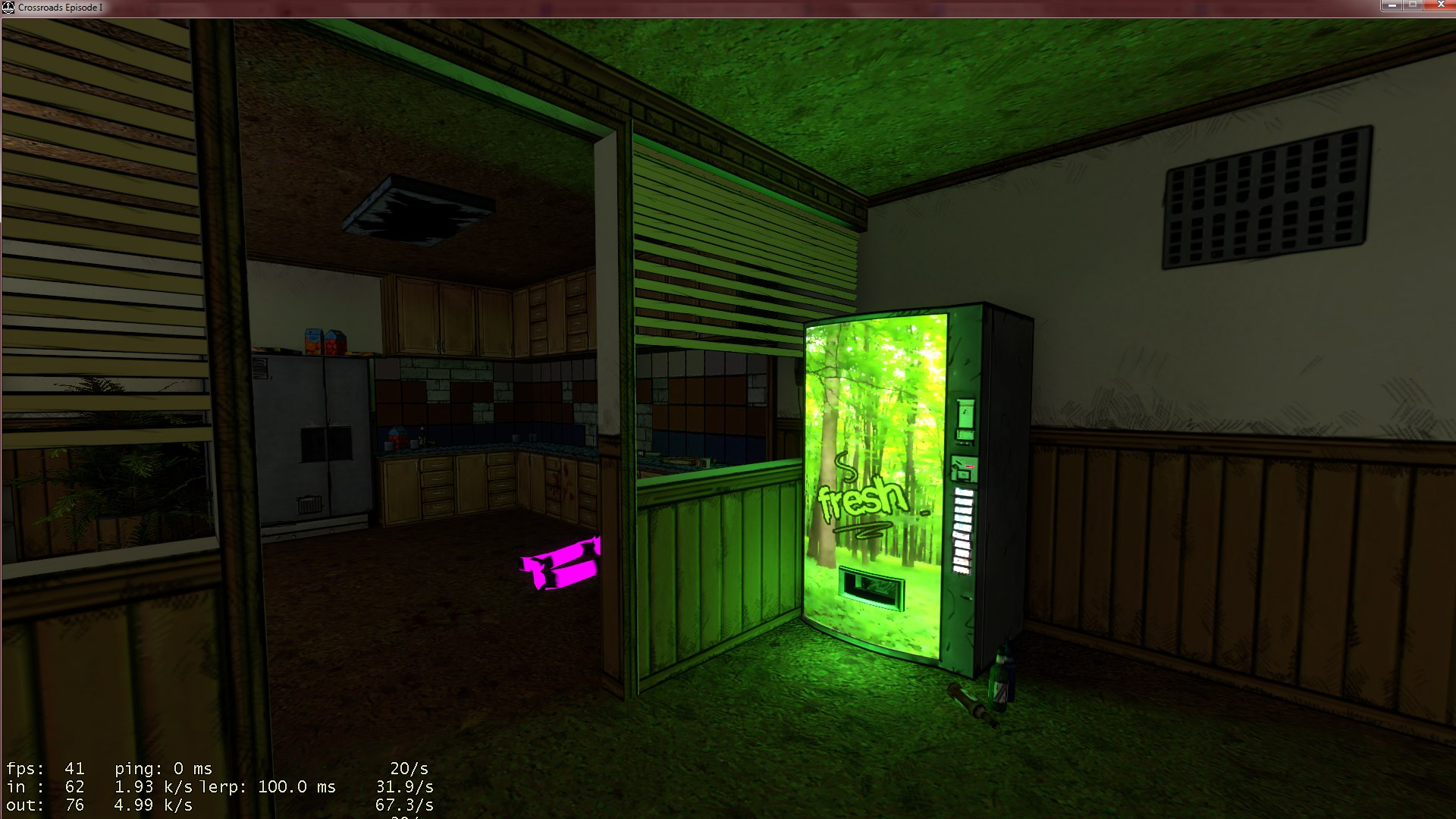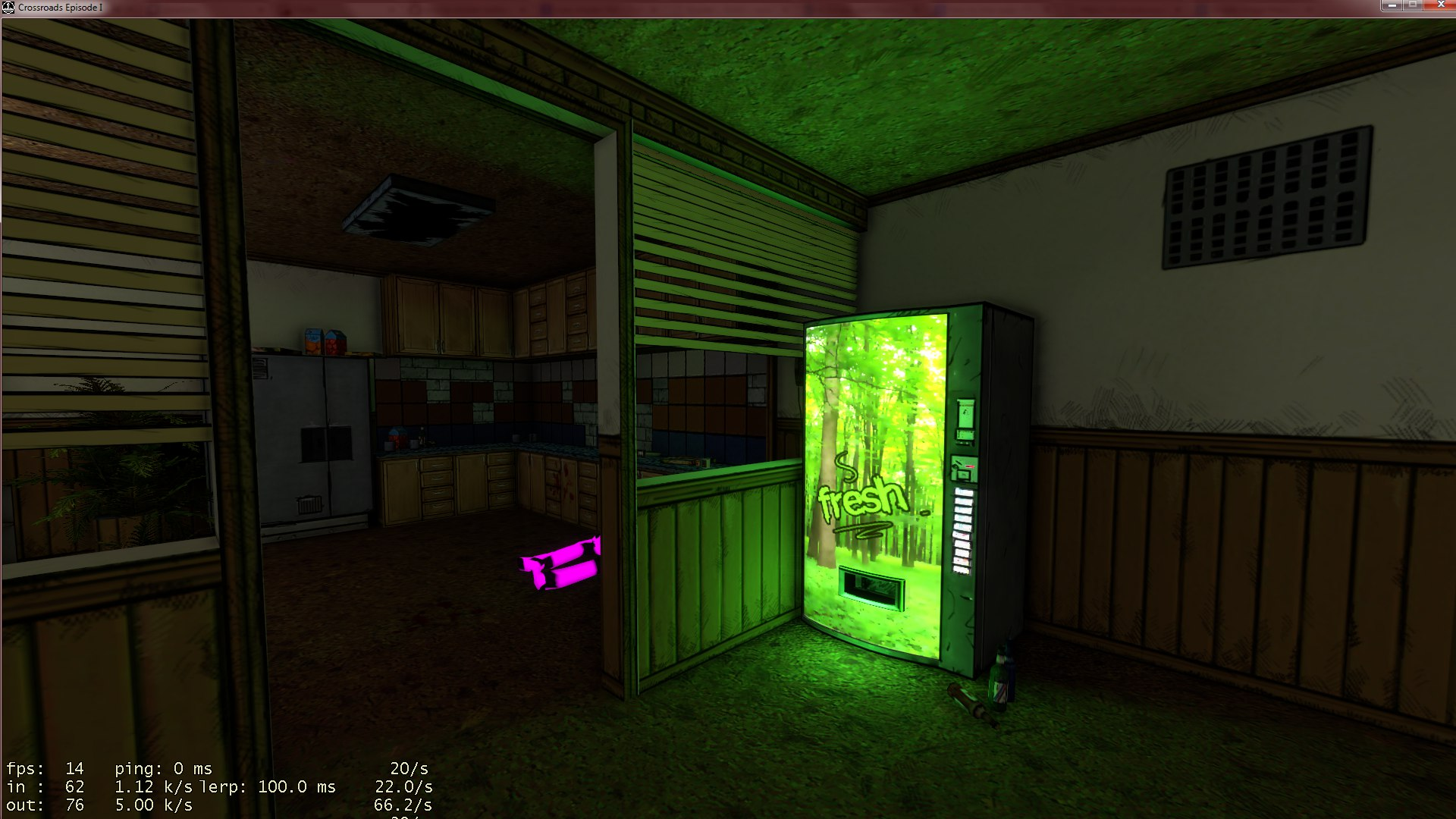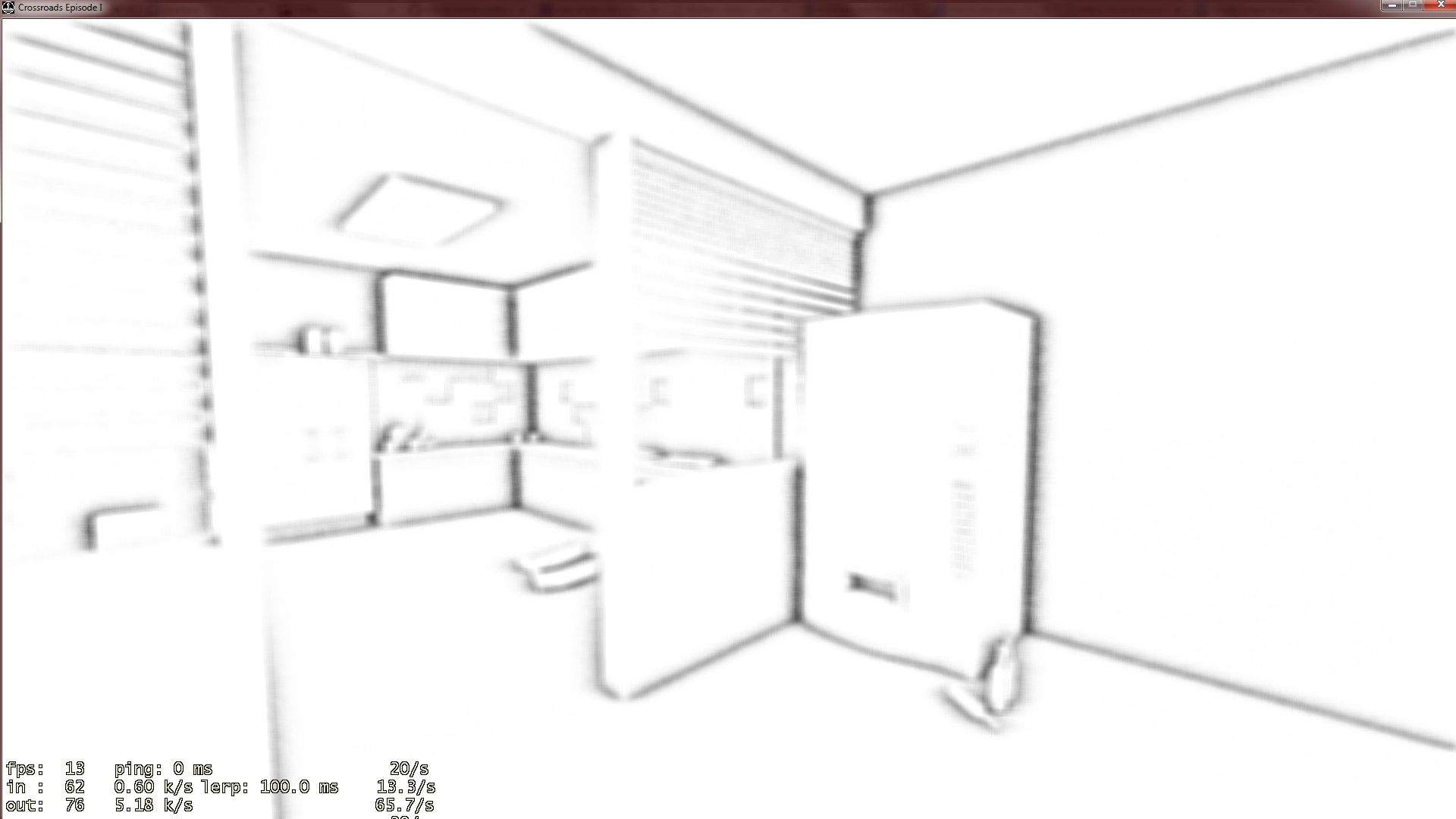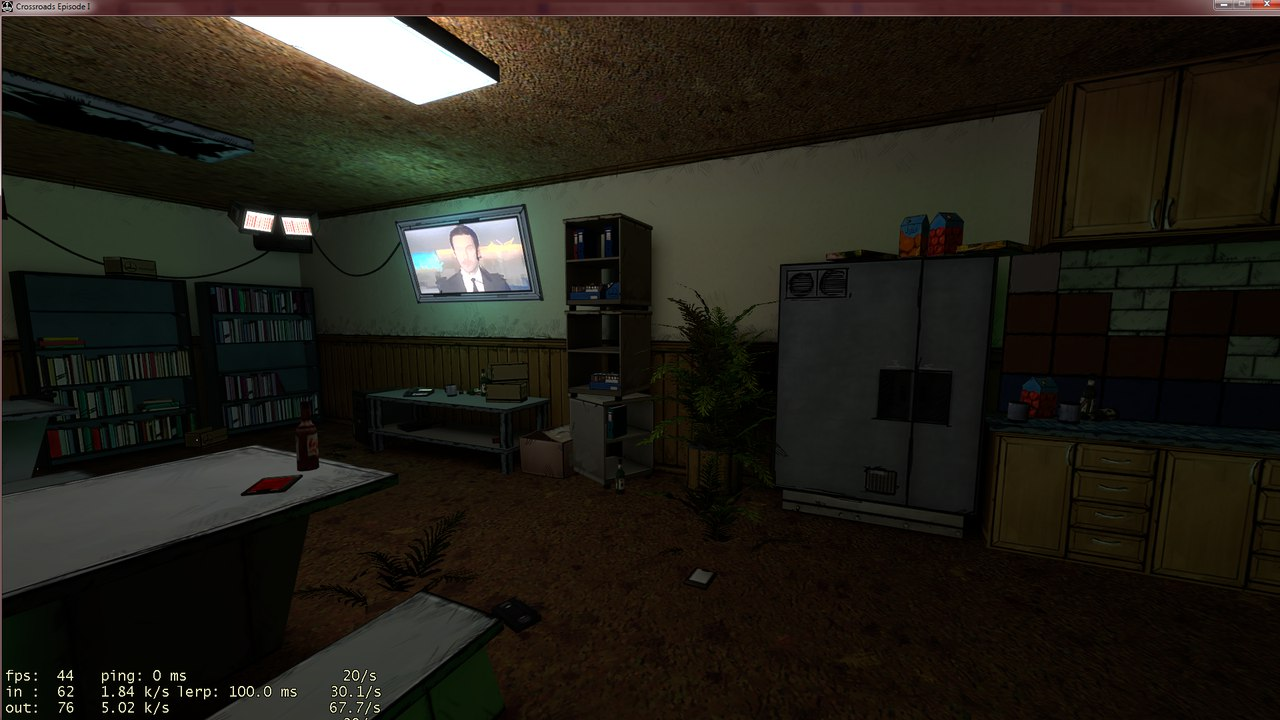[Source Engine] Implementing Basic SSAO Shader | Part 1

In this tutorial I will show you (not teach) how to implement basic SSAO shader into Source Engine.

Posted by on - Intermediate Client Side Coding

Hello everyone! This is my first tutorial about how to implement simple SSAO shader into Source Engine. That was my first experience with shaders, I implemented it in Crossroads, but it wasn't good enough and I replaced it with more advanced SSAO. As for this old version, it was finished and I just didn't want to delete it, so I decided to post it here.

Note: You won't get good SSAO effect with this shader, but you can use it as a base for futher improvements.

This is going to be simple copy-paste tutorial without much explanations and you probably have to read this Shader Authoring article before we get started. Also if you have found mistakes or have any questions fell free to ask me them in the comments.

This tutorial is split in two parts: first - implementing shader, second - making it work in game.

So, here we go. This is how it looks in Crossroads.Without SSAOWith SSAOSSAO pass with simple gaussian 3x3 blur (not depth blur)

The shader code itself is based on Arkano22's glsl shader for Blender. I ported it to Source with my custom additions. Also, the same shader currently ships with the Source Shader Editor.

Here is the code. Lets name it 'ssao_ps30.fxc':

``````// Crossroads SSAO Shader based off Arkano22's GLSL Shader ( assembled by Martins Upitis )
#include "common_ps_fxc.h"

sampler			FrameBufferSampler				: register( s0 );

const float2	g_TexelSize						: register( c0 );
const int		g_SamplesCount					: register( c1 );	// samples count
const float		g_SSAO_Bias						: register( c3 );	// self-shadowing reduction
const float		g_lumInfluence					: register( c4 );	// how much luminance affects occlusion
const float		g_SSAOContrast					: register( c5 );
const float		g_SSAOZNear						: register( c6 );	// Z-near
const float		g_SSAOZFar						: register( c7 );	// Z-far
const float		g_SSAO_Bias_Offset				: register( c8 );

#define PI		3.14159265

static float gdisplace = g_SSAO_Bias;	// gauss bell center

float2 rand(float2 coord, float2 size) //generating noise texture for dithering
{
float noiseX = frac(sin(dot(coord, float2(12.9898,78.233))) * 43758.5453) * 2.0f-1.0f;
float noiseY = frac(sin(dot(coord, float2(12.9898,78.233)*2.0)) * 43758.5453) * 2.0f-1.0f;

return float2(noiseX,noiseY)*0.001;
}

float readDepth(in float2 coord, sampler tex)
{
return tex2Dlod(tex, float4(coord, 0, 0)).a * (g_SSAOZNear / g_SSAOZFar);
}

float compareDepths(in float depth1, in float depth2,inout int far)
{
float garea = 1.0; //gauss bell width
float diff = (depth1 - depth2)*100.0; //depth difference (0-100)

//reduce left bell width to avoid self-shadowing
if ( diff < gdisplace + g_SSAO_Bias_Offset )
{
garea = g_SSAO_Bias;
}
else
{
far = 1;
}

float gauss = pow(2.7182,-2.0*(diff-gdisplace)*(diff-gdisplace)/(garea*garea));
return gauss;
}

float calAO(float2 uv, float depth, float dw, float dh, sampler tex)
{

float temp = 0.0;
float temp2 = 0.0;
float coordw = uv.x + dw*dd;
float coordh = uv.y + dh*dd;
float coordw2 = uv.x - dw*dd;
float coordh2 = uv.y - dh*dd;

float2 coord = float2(coordw , coordh);
float2 coord2 = float2(coordw2, coordh2);

int far = 0;
//DEPTH EXTRAPOLATION:
if (far > 0)
{
temp += (1.0-temp)*temp2;
}

return temp;
}

void DoSSAO( in float2 uv, in float2 texelSize, in sampler color_depth, out float ao_out )
{
float2 size = 1.0f / texelSize;
float2 noise = rand(uv,size);

float w = texelSize.x/clamp(depth, 0.25f, 1.0)+(noise.x*(1.0f-noise.x));
float h = texelSize.y/clamp(depth, 0.25f, 1.0)+(noise.y*(1.0f-noise.y));

float pw;
float ph;

float ao = 0;

float dl = PI*(3.0-sqrt(5.0));
float dz = 1.0/float( g_SamplesCount );
float z = 1.0 - dz/1.0;
float l = 0.0;

for (int i = 1; i <= g_SamplesCount; i++)
{
float r = sqrt(1.0-z);

pw = cos(l)*r;
ph = sin(l)*r;
ao += calAO( uv, depth, pw*w, ph*h, color_depth );
z = z - dz;
l = l + dl;
}
ao /= float( g_SamplesCount );
ao = 1.0-ao;

float3 color = tex2D(color_depth,uv).rgb;

float3 lumcoeff = float3( 0.2126f, 0.7152f, 0.0722f );
float lum = dot( color.rgb, lumcoeff );
float3 luminance = float( lum );

ao_out = lerp( ao, 1.0f, luminance*g_lumInfluence );
ao_out = ((ao_out - 0.5f) * max(g_SSAOContrast, 0)) + 0.5f;
}

struct PS_INPUT
{
HALF2 vTexCoord			:	TEXCOORD0;
};

float4 main( PS_INPUT i ) : COLOR
{
float ssao_out = (float)0;
DoSSAO( i.vTexCoord, g_TexelSize, FrameBufferSampler, ssao_out );

float3 out_col = float( ssao_out.x );
out_col = out_col * float( 2.0 );

return float4( out_col, 1.0f );
}``````

Lets name it 'ssao_vs30.fxc':

``````#include "common_vs_fxc.h"

struct VS_INPUT
{
float4 vPos			: POSITION;
float2 vTexCoord	: TEXCOORD0;
};

struct VS_OUTPUT
{
float4 vProjPos			: POSITION;
float2 vTexCoord		: TEXCOORD0;
};

VS_OUTPUT main( const VS_INPUT v )
{
VS_OUTPUT o = ( VS_OUTPUT )0;

o.vProjPos = float4( v.vPos.xyz, 1.0f );
o.vTexCoord = v.vTexCoord;

return o;
}``````

You can compile them now without any errors.

g_TexelSize - contains two variables: 1/render target width and 1/render target height;

g_SamplesCount - is ssao samples. More samples - more realistic ssao, but also more expensive;

g_SSAO_Radius - is the size of ssao effect;

g_SSAO_Bias - is ssao 'accuracy'. Too accurate ssao can cause artifacts (shadows) on flat surfaces. This variable is needed to reduce such artifacts;

g_lumInfluence - is how strong ambient light affects on ssao. The brighter light in the room the less ssao you will see;

g_SSAOContrast - is how strong the ssao effect (how dark is ssao shadows);

g_SSAOZNear - as far as I remember, minimum distance for object to be close to another object to cast shadows;

g_SSAOZFar - as far as I remember, maximum distance for object to be close to another object to cast shadows;

g_SSAO_Bias_Offset - as far as I remember, you shouldn't change this;

Okay, now we have the ssao shader, but we need another shader that is going to combine ssao pass and regular framebuffer:

It is the same as ssao vertex shader. Lets name it 'ssao_combine_vs30'.

Lets name it 'ssao_combine_ps30':

``````#include "common_ps_fxc.h"

sampler ssaosampler			: register( s0 );
sampler framebuffersampler		: register( s1 );

struct PS_INPUT
{
HALF2 vTexCoord			:	TEXCOORD0;
};

float4 main( PS_INPUT i ) : COLOR
{
float4 framebuftex = tex2D( framebuffersampler, i.vTexCoord );
float3 framebufcol = framebuftex.rgb;
float framebufalpha = framebuftex.a;
float ssaotex = tex2D( ssaosampler, i.vTexCoord ).r;

framebufcol = framebufcol * ssaotex;

return float4( framebufcol, framebufalpha );
}``````

Okay, thats all with shaders! In the next part I will show you how to make it work in game and say some words about blur.

Another screenshot with and witout ssao:Without SSAOWith SSAO

Feel free to ask me questions or suggest fixes for shader or tutorial. Hope you will find this tutorial useful.

Have a nice day! The next part is here.

Fantastic, pushing the old source engine to new heights!

Looks great good luck in the future!

It actually makes a big difference to such an old engine. Keep up the great work!

Noice!

nice but your fps drops more than half with ssao and i'm guessing you have a decent setup?

That shots were taken on my laptop with GeForce GTX 650m at 1080p. The SSAO settings were maxed out, but you can tweak them and find the balance between fps and quality. SSAO is quite expensive shader itself, even more in Source.

Does this work on AMD cards?

It should, but we didn't test it

Thanks! That's an awesome tutorial!
When do you plan on releasing part 2? Can't wait :)

In a few days. Maybe today.

Damn, I'd love to see it today. I'm so excited!

I a little updated this tutorial and also added link to the second part.

why it say 40 fps for without and less than 20 for with

noticed also on first shots , same type of fps, if there is some env_projected texture and 40k vertex

Question, this is for the 2013 branch correct?

yep, but it can work on both 2010 & 2013 branches.